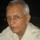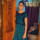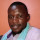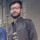1. Home >
2. Apps >
3. Groups >

# Difference between load and resistor

Question asked by sayandev in #Coffee Room on Nov 15, 2010sayandev · Nov 15, 2010
Rank D2 - MASTER
We know that a resistor can be considered as a load. Now the question is, if we increase the resistance according to ohm's law V = IR, the current must fall. Again we say that if the load is increased in the circuit, current consumption gets higher. So the question is, what happens to the circuit current if the load is increased or decreased?? Posted in: #Coffee Roomaj_onduty · Nov 16, 2010
Firstly you have to consider the fact that the Ohm's law graph is not a straight line when we apply practically.
Secondly, you have to understand the fact that the load what we are discussing here is not always the resistance, the load may be capacitive or inductive, or even all the three. So, we cannot relate it with Ohm's law. To sum up, when the load increases, there is a voltage drop which leads to the increase in current, since power=volt*current*power factor.aj_onduty · Nov 16, 2010
Ohm's law is intended to define the resistance provided by the conductor as the ratio of the voltage applied to the current which flows through the conductor.
But I want more experts to talk about it here. Please do. I hope Voltaire would be watching this thread.
@sayandev, nice question! 👍sushant005 · Nov 16, 2010
Rank C1 - EXPERT
@ Aj_onduty: Very nice explanation.

The above point is very clear to distinguish the load and the resistance.sayandev · Nov 17, 2010
Rank D2 - MASTER
Thanks for the explanation. But the thing is that I know that a load can be resistive,inductive,capacitive or a mixing of these. My question is on the fact of change in current in the circuit when we increase load and when we increase impedence in the circuit.

@aj_onduty On the basis of your reply, I am asking you that is there any difference b/w impedence of the conductor and load ??

And please anyone explain me. I am still confused ???sayandev · Nov 17, 2010
Rank D2 - MASTER
"We know that a resistor can be considered as a load. Now the question is, if we increase the resistance according to ohm's law V = IR, the current must fall. Again we say that if the load is increased in the circuit, current consumption gets higher. So the question is, what happens to the circuit current if the load is increased or decreased??"sreeram peri · Nov 17, 2010
Rank E1 - BEGINNER
the thing is, "lLOAD IS NOT A RESISTOR" resistor a mere representation of a load... consider a practiacal xample of generator, in GENERATOR to generate electricity required to rotate all d fans in a function hall... now here OUTput of generator is electricity,which needs to be increased if number of fans to be rotated increases,here LOAD(fans) draw curent from generator,as load (no. of fans)increases,d generator needs t generate more n more eletricity to meet its demand... hence LOAD and CURRENT are directly proportionaL.. think practicallyaj_onduty · Nov 17, 2010
Have you heard of a potential drop?
When we connect a load to the terminals of a generator, what happens is that there is a potential drop across the load. To be in layman's terms, the generator gives and the load consumes. It has to consume the same amount of what the generator is giving.
Suppose we have two resistive loads for instance, R1 and R2 in series. The load should be equal to the total resistance R-
That is,
R=R1+R2------------------(1)
But we know V=IR.
Therefore,
R=V/I---------------------(2)
Similarly,
R1=V1/I1-----------------(3)
R2=V2/I2-----------------(4)
Put (2),(3),(4) in (1),
V/I=V1/I1+V2/I2
But I1=I2=I(Since the connection is in series)
Therefore, we get V=V1+V2.----------(5)(V1 and V2 are the voltage drops across the loads)
That means the whole voltage applied to the system is equal to the sum of the potential drops across the loads. A potential drop is basically equal to a battery connected upside down into the system.According to Kirchhoff's voltage law,the algebraic sum of the voltages in a circuit is zero. This is what I meant by proving the above equation number (5), which can be also said as-
V-V1-V2=0
So, when you go on connecting loads, the work as batteries. They pull more and more current into themselves and thus, during the addition of a load, the current increases.sayandev · Nov 18, 2010
Rank D2 - MASTER
Now suppose I double the load. then?? I think then the potential drop across the load must double, if the circuit current remains constant. But if we keep the voltage source constant, then the current must decrease... Is it so?? which case happens???aj_onduty · Nov 21, 2010
I saw this doubt right now, I am logged in through my mobile. As my phone does not permit moe than 512characters, i cannot type the answer.I will post the answer tomorrow at 10am! Thanks for asking!aj_onduty · Nov 21, 2010
Hi,
First, consider the fact that in a power system, the voltage cannot be changed.
Let me explain what happens in the power system. When there is no load in the system, the system does run, the power source(generator) supplies for the losses which are present in a power system.
You know the formula of power, for those who don't, P=VIcos(phi). Lets not consider about the cos phi part, as we are interested to know about the V and I in here.
So, the current value will be minimal when there is no load. Now, when you input any load into the system, you are putting in something which is power hungry, which presses your generator to input more power. So, the power in the system increases. That is, P increases. When P increases, V and I must also increase. But V cannot increased, as was mentioned before. So, I should be increased. Thus, when every unit of load is added, the current value increases. This never means that Ohm's law is not followed. The law is followed. but what happens is that the current decrease due to it does not match with the current increase due to increase in power.mehaque87 · Nov 24, 2010
Rank E2 - BEGINNER
when we increase load or when we increase impedence in the circuit for a particular voltage source as a i/p current will decrease .nsiva007 · Nov 25, 2010
Rank D2 - MASTER
Load by what yo ask is the current intake...a heavy load draws more current and less resistance for that matter... while a lighter load means less current drawn and more resistance...!sayandev · Nov 26, 2010
Rank D2 - MASTER
Thanks ajit.... then the question is that how everytime is the KVL (kirchoff's voltage law) maintained in the circuit??aj_onduty · Nov 27, 2010
I believe that all the laws defining the relation of current and voltage with each other or with something else or itself, like the KVL, KCL, Ohm's law follow one basic assumption, the power applied in the circuit is kept constant. Hope I am clear. Hope I am correct. Correct me if I am wrong, Thank you.sayandev · Nov 27, 2010
Rank D2 - MASTER
Got it...😛.. Now its clear.....HIMAYUN KHAN 1560712 · Dec 18, 2018
Rank E1 - BEGINNER

i am still confused...what is the difference between load and resistance??

and what is resistance....is it positively charge or negatively charged substance?

note: if we dont follow ohm"s law in transmission power. then what is ohms law and why its so famous....Ramani Aswath · Dec 18, 2018
Rank A1 - PROKaustubh Katdare · Dec 19, 2018
Rank A1 - PRO

@HIMAYUN KHAN 1560712 - In electric / electronic circuits; a load is anything that draws current from the circuit. Typically, the household appliances are considered as 'load'; and it's still a 'resistance'.Kavya Burramolla · Feb 4, 2020
Rank C1 - EXPERT

Load can be anything not specifically a resistor. Basically the device that draws or consumes the current from the circuit is considered to be a load. In practical sense resistor is an device which opposed the flow of current which mean when resistance value gos high the current through the circuit will be minimal. Higher the resistance lower the current flow. Resistor observes the flow of current and emits in the form of heat. But the load is something which is utilising the current flow through the circuit and working as per the application. The load can be fan, TV, other home appliances.bill yorke · Feb 6, 2020
Rank C2 - EXPERT

Resistance is the common term you've heard of everywhere. Constant proportionality from law of ohm.
Load resistance is still a resistance, however in the sense of electrical circuits it has a very specific definition; it is the resistance linked between a circuit's output point, and the ground.
This resistance takes from the circuit in the power provided.

Such resistance often means the system or circuit resistance that would be related to the exit level. So our ceiling fans, tubelights, toasters, room heaters, etc. are all considered by the mains circuit as load resistance (more suitably, load impedance).Durgesh Srivastava · Feb 20, 2020
Rank C1 - EXPERT

Resistor observes the flow of current and emits in the form of heat. But the load is something which is utilising the current flow through the circuit and working as per the application. Load resistance is still a resistance.Vikrant Singh · May 15, 2020
Rank E2 - BEGINNER

" load is something which is utilising the current flow". how is "utilizing the current" different from emitting heat? what exactly is utilizing the current flow?

for eg, is it the capacitor storing the charge in a circuit, thereby demanding more current from the circuit?

## You must log-in or sign-up to reply to this post.

Click to Log-In or Sign-Up